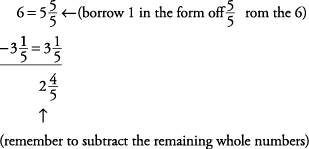## Adding and Subtracting Mixed Numbers

You will notice that the steps in adding and subtracting mixed numbers are similar. But also note the differences and the reminders given in this section.

To add mixed numbers, the same rule as in adding fractions applies (find the LCD), but make sure that you always add the whole numbers to get your final answer.

Add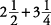.Sometimes, you may end up with a mixed number that includes an improper fraction. In that case, you must change the improper fraction to a mixed number and combine it with the sum of the integers.

Add.When you subtract mixed numbers, you sometimes may have to rename the whole number, just as you sometimes borrow from the next column when subtracting whole numbers. Note: When you borrow 1 from the whole number, the 1 must be changed to a fraction.

Subtract

651 – 129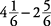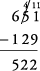(You borrowed 1 from the 10's column.)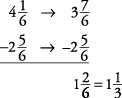(You borrowed 1 in the form offrom the 1's column.)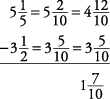(You borrowed 1 in the form offrom the 1's column.)

Notice that you should borrow only after you have identified a common denominator.

To subtract a mixed number from a whole number, you have to borrow from the whole number.

Subtract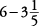.